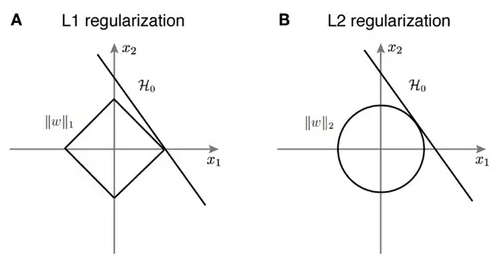## When would you choose L1-norm vs. L2-norm?

• In a typical setting, the L2-norm is better at minimizing the prediction error over the L1-norm. However, we do find the L1-norm being used despite the L2-norm outperforming it in almost every task, and this is primarily because the L1-norm is capable of producing a sparser solution.

• To understand why an L1-norm produces sparser solutions over an L2-norm during regularization, we just need to visualize the spaces for both the L1-norm and L2-norm.• In the diagram above, we can see that the solution for the L1-norm (to get to the line from inside our diamond-shaped space), it’s best to maximize $$x_1$$ and leave $$x_2$$ at $$0$$, whereas the solution for the L2-norm (to get to the line from inside our circular-shaped space), is a combination of both $$x_1$$ and $$x_2$$. It is likely that the fit for L2 will be more precise, however, with the L1 norm, our solution will be more sparse.

• Since the L2-norm penalizes larger errors more strongly, it will yield a solution which has fewer large residual values along with fewer very small residuals as well.

• The L1-norm, on the other hand, will give a solution with more large residuals, however, it will also have a lot of zeros in the solution. Hence, we might want to use the L1-norm when we have constraints on feature extraction. We can easily avoid computing a lot of computationally expensive features at the cost of some of the accuracy, since the L1-norm will give us a solution which has the weights for a large set of features set to $$0$$. A use-case of L1 would be real-time detection or tracking of an object/face/material using a set of diverse handcrafted features with a large margin classifier like an SVM in a sliding window fashion, where you would probably want feature computation to be as fast as possible in this case.

• Another way to interpret this is that L2-norm basically views all features similarly since the “distance” between features is similar (given its geometric representation as a circle), while L1 norm treats different features differently. Thus, if you are unsure of the kind of features available in your dataset and their relative importance, L2 regularization is the way to go. On the other hand, if you know that one feature matters much more than another, use L1 regularization.

• In summary,

• Broadly speaking, L1 is more useful for “what?” and L2 is more “how much?”.
• The L2 norm is as smooth as your floats are precise. It captures energy and Euclidean distance, things you want when, for e.g., tracking features. It’s also computation heavy compared to the L1 norm.
• The L1 norm isn’t smooth, which is less about ignoring fine detail and more about generating sparse feature vectors. Sparse is sometimes good e.g., in high dimensional classification problems.

Here are some (optional) links you may find interesting for further reading:

## Citation

If you found our work useful, please cite it as:

@article{Chadha2020DistilledNorm,
title   = {L1 vs. L2 Norm},